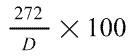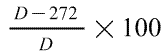xmlns:atom="http://www.w3.org/2005/Atom" xmlns:atom="http://www.w3.org/2005/Atom"

# SCHEDULES

## SCHEDULE 4U.K.Pensions: annual allowance

### PART 3 U.K.Calculation of pension input amounts for periods ending in 2015-16

7U.K.Part 4 of FA 2004 is amended as follows.

8U.K.In section 229 (total pension input amount), after subsection (4) insert—

(5)Subsection (2) is subject to section 237ZA (calculation of pension input amounts for input periods ending in 2015-16).

9U.K.After section 237 insert—

#### “237ZAPension input amounts for input periods ending in 2015-16

(1)This section applies where the tax year is the pre-alignment tax year or the post-alignment tax year (see section 228C(2)).

##### Modified rules for cash balance, or defined benefits, arrangement

(2)The rules for calculating the pension input amount in respect of a cash balance arrangement, or a defined benefits arrangement, are modified as follows (and the rules for calculating the pension input amount in respect of a hybrid arrangement have effect accordingly).

##### Single input amount to be calculated for combined period

(3)The pension input amount in respect of the arrangement is the time-apportioned percentage of any increase in the value of the individual's rights under the arrangement during the period (“the combined period”) that consists of the combination of all pension input periods of the arrangement that end—

(a)on or after 6 April 2015 but on or before 8 July 2015, or

(b)on 5 April 2016.

(4)To calculate the increase (if any) in the value of the individual's rights under the arrangement during the combined period, apply (as the case may be) sections 230 to 232 (except section 230(1)), or sections 234 to 236A (except section 234(1)), as if—

(a)references to the pension input period were references to the combined period,

(b)the combined period were a pension input period of the arrangement,

(c)2.5% were the appropriate percentage specified in section 231(3) or 235(3), and

(d)2.5% were the percentage mentioned in paragraph (c) of the definition of “relevant percentage” given by section 230(5C) or 234(5C),

but paragraph (d) does not have effect for the purposes of the definition of “CPI percentage” given by section 234(5C).

##### Apportioning input amount for combined period to tax years

(5)“The time-apportioned percentage” for the post-alignment tax year is—and “the time-apportioned percentage” for the pre-alignment tax year is—where D is the number of days in the combined period.

##### Calculation and apportionment rules modified in certain cases

(6)Subsections (3) to (5) have effect subject to the following provisions of this section.

##### Exceptions in certain cases where individual is deferred member of scheme

(7)Subsections (3) to (5) do not apply, and subsections (8) and (9) apply instead, if—

(a)because of section 238ZA(2), a pension input period for the arrangement ends with 8 July 2015,

(b)another pension input period for the arrangement ends with a day (“the unchanged last day”) after 5 April 2015 but before 8 July 2015, and

(c)section 230(5B) or 234(5B), when applied separately to each of—

(i)the pension input period for the arrangement ending with 8 July 2015, and

(ii)the pension input period for the arrangement ending with 5 April 2016,

gives the result that the pension input amount in respect of the arrangement for each of those periods is nil.

(8)The pension input amount in respect of the arrangement for the post-alignment tax year is nil.

(9)The pension input amount in respect of the arrangement for the pre-alignment tax year is the amount which would be the pension input amount in respect of the arrangement for the pre-alignment tax year if—

(a)the pension input period ending with the unchanged last day were the only pension input period for the arrangement ending in the pre-alignment tax year, and

(b)subsections (3) to (5) were ignored.

##### Modifications in some other cases where individual is deferred member of scheme

(10)Subsections (11) to (13) apply if—

(a)because of section 238ZA(2), a pension input period for the arrangement ends with 8 July 2015,

(b)apart from section 238ZA(2), that pension input period (“the cut-short period”) would have ended with a day (“the original last day”) after 8 July 2015 but before 5 April 2016,

(c)at or after the beginning of the cut-short period but not later than the original last day, or in an earlier pension input period for the arrangement, the individual becomes a deferred member of the pension scheme that the arrangement is under, and

(d)were the period—

(i)beginning with the day after the original last day, and

(ii)ending with 5 April 2016,

a pension input period for the arrangement, the pension input amount in respect of the arrangement for that period would be nil by virtue of section 230(5B) or 234(5B).

(11)Subsections (3) to (5) have effect as if the original last day, and not 5 April 2016, were the last day of the combined period (so that, in particular, D in subsection (5) is the number of days in the combined period as so shortened).

(12)If the individual becomes a deferred member of the pension scheme in a pension input period for the arrangement earlier than the cut-short period—

(a)the time-apportioned percentage for the post-alignment tax year is treated as being nil, and

(b)the time-apportioned percentage for the pre-alignment tax year is treated as being 100.

(13)If the individual becomes a deferred member of the pension scheme at or after the beginning of the cut-short period but not later than the original last day, subsection (5) has effect as if for “ 272 ”, in each place, there were substituted the number of days in the period beginning with 9 July 2015 and ending with the original last day.

##### Modification where first input period ends with 5 April 2016

(14)If the first pension input period for the arrangement ends with 5 April 2016—

(a)the time-apportioned percentage for the post-alignment tax year is treated as being 100, and

(b)the time-apportioned percentage for the pre-alignment tax year is treated as being nil.

##### Modification where last input period ends before 9 July 2015

(15)If the last pension input period for the arrangement ends after 5 April 2015 but before 9 July 2015—

(a)the time-apportioned percentage for the post-alignment tax year is treated as being nil, and

(b)the time-apportioned percentage for the pre-alignment tax year is treated as being 100.

##### Alternative modifications where individual is deferred member of scheme

(16)Subsections (17) and (18) apply if—

(a)subsections (8) and (9) do not apply,

(b)subsections (11) to (13) do not apply,

(c)subsection (14) does not apply, and

(d)section 230(5B) or 234(5B), when applied separately to each of—

(i)so much of the combined period as consists of the post-alignment tax year, and

(ii)the remainder of the combined period (for this purpose treating that remainder as a single pension input period if not otherwise the case),

gives the result that the pension input amount in respect of the arrangement for one (but not the other) of those parts of the combined period is nil.

(17)If the nil result is for so much of the combined period as consists of the post-alignment tax year—

(a)the time-apportioned percentage for the post-alignment tax year is treated as being nil, and

(b)the time-apportioned percentage for the pre-alignment tax year is treated as being 100.

(18)If the nil result is for so much of the combined period as precedes 9 July 2015—

(a)the time-apportioned percentage for the pre-alignment tax year is treated as being nil, and

(b)the time-apportioned percentage for the post-alignment tax year is treated as being 100.